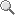### <!-- function zoomIn() { newZoom= parseInt(oZoom.style.zoom)+10+'%' oZoom.style.zoom =newZoom; } function zoomOut() { newZoom= parseInt(oZoom.style.zoom)-10+'%' oZoom.style.zoom =newZoom; } //--> <!-- function MM_swapImgRestore() { //v3.0 var i,x,a=document.MM_sr; for(i=0;a&&i<a.length&&(x=a[i])&&x.oSrc;i++) x.src=x.oSrc; } function MM_preloadImages() { //v3.0 var d=document; if(d.images){ if(!d.MM_p) d.MM_p=new Array(); var i,j=d.MM_p.length,a=MM_preloadImages.arguments; for(i=0; i<a.length; i++) if (a[i].indexOf("#")!=0){ d.MM_p[j]=new Image; d.MM_p[j++].src=a[i];}} } function MM_findObj(n, d) { //v4.01 var p,i,x; if(!d) d=document; if((p=n.indexOf("?"))>0&&parent.frames.length) { d=parent.frames[n.substring(p+1)].document; n=n.substring(0,p);} if(!(x=d[n])&&d.all) x=d.all[n]; for (i=0;!x&&i<d.forms.length;i++) x=d.forms[i][n]; for(i=0;!x&&d.layers&&i<d.layers.length;i++) x=MM_findObj(n,d.layers[i].document); if(!x && d.getElementById) x=d.getElementById(n); return x; } function MM_swapImage() { //v3.0 var i,j=0,x,a=MM_swapImage.arguments; document.MM_sr=new Array; for(i=0;i<(a.length-2);i+=3) if ((x=MM_findObj(a[i]))!=null){document.MM_sr[j++]=x; if(!x.oSrc) x.oSrc=x.src; x.src=a[i+2];} } //-->来自翠苑一小五年级的傅智豪小朋友在爸爸的抱举下写下了新年祝福。昨晚，杭州雷峰塔景区以登高、祈福、感恩为主题，邀请了十多户家庭齐聚雷峰塔下，一起登高祈福，在祝福墙上写上新年的祝福，体验印度抬头见喜、匈牙利抢喇叭送祝福、西班牙听钟声吃葡萄等世界各地的元旦互动活动。

 请您文明上网、理性发言并遵守相关规定，在注册后发表评论。 只有浙江在线注册用户可以发表评论...... 我要注册最热新闻民生帮帮帮推荐专题